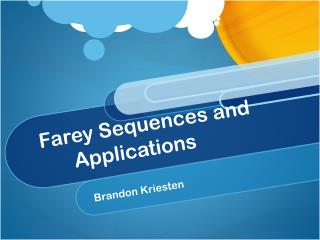# Farey Sequences and Applications - PowerPoint PPT PresentationDownload PresentationFarey Sequences and Applications

Farey Sequences and ApplicationsDownload Presentation## Farey Sequences and Applications

- - - - - - - - - - - - - - - - - - - - - - - - - - - E N D - - - - - - - - - - - - - - - - - - - - - - - - - - -
##### Presentation Transcript

1. Farey Sequences and Applications Brandon Kriesten

2. Outline • Definition of a Farey Sequence • Properties of Farey Sequences • Construction of the Farey Sequence • Theorem of two successive elements • Number of Terms in a Farey Sequence • Applications of Farey Sequences • Ford Circles

3. Definition of a Farey Sequence • A Farey Sequence is defined as all of the reduced fractions from [0,1] with denominators no larger than n, for all n in the domain of counting numbers, arranged in the order of increasing size.

4. Some Examples of Farey Sequences • F_1 = {0/1, 1/1} • F_2 = {0/1, 1/2, 1/1} • F_3 = {0/1,1/3,1/2,2/3,1/1} • F_7 = {0/1, 1/7, 1/6, 1/5, 1/4, 2/7, 1/3, 2/5, 3/7, 1/2, 4/7, 3/5, 2/3, 5/7, 3/4, 4/5, 5/6, 6/7, 1/1}

5. How to Construct the Farey Sequence • To construct the Farey Sequence we must understand the concept of the mediant property. • The mediant property is stated as follows: Given a Farey Sequence F_(n) with the adjacent elements a/b < c/d, in the sequence F_(n+1) the elements will be mediated by the construction a/b < (a+c)/(b+d) < c/d if b+d ≤ n. If b+d > n the number will appear in a later sequence. Now from this definition and given F_2, construct F_3 and F4.

6. Theorem Theorem: Given a Farey sequence F_(n) with two successive elements (p_1)/(q_1) and (p_2)/(q_2), where (p_2)/(q_2) > (p_1)/(q_1), show that (p_2)/(q_2) - (p_1)/(q_1) = 1/(q_1*q_2).

7. Euler’s Totient Function • To construct an equation for the number of elements in a Farey Sequence, we must first understand the concept of Euler’s Totient Function. Euler’s Totient function represents the number of positive integers less than a number n that are coprime to n. It is represented as the Greek symbol “phi” φ. • E.g. φ(24) = 8, φ(6) = 2.

8. The number of Terms in a Farey Sequence • The number of terms in a Farey Sequence is given by 1 plus the summation from k=1 to “n” of Euler’s Totient function. • This can also be written as the number of elements in F_(n-1) + φ(n).

9. The Ford Circle

10. Ford Circles cont… • A Ford Circle is defined as: For every rational number p/q where gcd(p,q) = 1, there exists a Ford Circle C(p,q) with a center (p/q, 1/2q^2) and a radius of 1/2q^2, that is tangent to the x axis at the point (p/q, 0).

11. Theorem • Theorem: The Largest Ford circle between tangent Ford circles. Suppose that C(a, b) and C(c, d) are tangent Ford circles. Then the largest Ford circle between them is C(a + c, b + d), the Ford circle associated with the mediant fraction.

12. For Further Research • http://mathworld.wolfram.com/FareySequence.html • http://golem.ph.utexas.edu/category/2008/06/farey_sequences_and_the_sternb.html • http://math.stanford.edu/circle/FareyFord.pdf